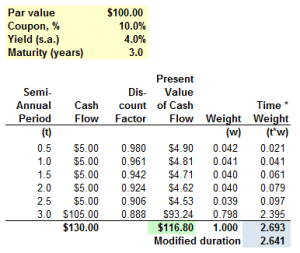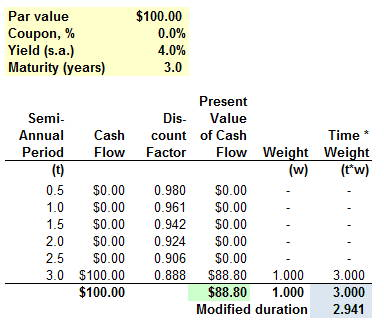# Blog Article

Stay in the know with all things Bionic Turtle

## Modified Duration

Our forum contains hundreds of questions about duration. Duration often vexes new candidates, in part because there are several types. Let’s first settle a confusion: the units of all durations are years (time). So, regardless of which duration, we can typically say something like “The bond’s duration is 4.52 years.” I taught duration several years under the popular but mistaken assumption that modified duration was unitless, while Macaulay duration was expressed in time. But years ago a customer schooled me with the math (paid members can view here) and showed me why all durations are expressed in units of time.

### Borrowing From CFA’s Definitions

Let’s see how the CFA curriculum introduces modified duration (I was curious, aren’t you?):

“The duration of a bond measures the sensitivity of the bond’s full price (including accrued interest) to changes in the bond’s yield-to-maturity or, more generally, to changes in benchmark interest rates. Duration estimates changes in the bond price assuming that variables other than the yield-to-maturity or benchmark rates are held constant. Most importantly, the time-to-maturity is unchanged. Therefore, duration measures the instantaneous (or, at least, same-day) change in the bond price. The accrued interest is the same, so it is the flat price that goes up or down when the full price changes. Duration is a useful measure because it represents the approximate amount of time a bond would have to be held for the market discount rate at purchase to be realized if there is a single change in interest rate. If the bond is held for the duration period, an increase from reinvesting coupons is offset by a decrease in price if interest rates increase and a decrease from reinvesting coupons is offset by an increase in price if interest rates decrease. There are several types of bond duration. In general, these can be divided into yield duration and curve duration. Yield duration is the sensitivity of the bond price with respect to the bond’s own yield-to-maturity. Curve duration is the sensitivity of the bond price (or more generally, the market value of a financial asset or liability) with respect to a benchmark yield curve. The benchmark yield curve could be the government yield curve on coupon bonds, the spot curve, or the forward curve, but in practice, the government par curve is often used. Yield duration statistics used in fixed-income analysis include Macaulay duration, modified duration, money duration, and the price value of a basis point (PVBP). A curve duration statistic often used is effective duration.” — source: CFA Institute. 2015 CFA Level I Volume 5 Equity and Fixed Income. Wiley Global Finance, 2014-07-14.

I think this definition is helpful for at least two points:

1. It highlights how duration-as-time is linked to duration’s essential purpose as a measure of bond risk (and specifically, the market risk of a bond).
2. It reminds us that, while duration can utilize different risk factors, the most common is the bond’s yield to maturity (aka, yield).

### An Example to Illustrate Modified Duration

Let’s assume a \$100.00 face value bond with three years to maturity that pays semi-annual coupons at a coupon rate of 10.0%. Further, let’s assume the bond’s yield-to-maturity (yield) is 4.0% per annum with semi-annual compounding:Macaulay duration has a convenient definition: Macaulay duration is the weighted average maturity of a bond. In our example, the bond has a price of \$116.80 (did you expect it to price above par because the yield is less than the coupon? Fantastic!). The bond’s final cash flow of \$105.00 has a present value of \$93.24 because the 3-year discount factor is 0.888 = (1+ + 0.06/2)^-(3*2); i.e., the present value of one dollar discounted at 4.0% semi-annually is \$0.888. The weight of this cash flow, as a percentage of the bond’s price, is 0.798 or 79.82%; that is, \$93.24/\$116.80 = 79.82%. The final column multiplies the time period by this weight; e.g., 0.798 * 3.0 years = 2.395 years (last row before the summation). The weighted average maturity of this bond (where the weights are the present values of the bond’s cash flows given as a proportions of the bond’s price) is 2.693 years.

If our yield were instead 4.0% per annum with continuous compounding, then this bond’s modified duration would also be 2.693 years. That’s because the relationship between these two durations (which an FRM® candidate should memorize) is given by: modified duration*(1+yield/k) = Macaulay duration, where (k) is the number of compound periods per year. Therefore, modified duration = Mac duration/(1+yield/k); i.e., for any discrete compound frequency, modified duration is a little less than Macaulay duration. But for continuous compounding, they are the same as (1+yield/k) tends to 1.0 as k tends to infinity.

Our bond has a semi-annual (k = 2) yield of 4.0% so it’s modified duration equals 2.641 years = 2.693 * (1+0.04/2).

### How can we interpret this?

Duration is a function of the bond’s first partial derivative with respect to yield. We use it to approximate the bond’s exposure to interest rate risk, which is a type of market risk. The keyword “approximation” is important.

Here are two interpretations of this bond’s modified duration of 2.641 years:

• If yield increases by 100 basis points (1.0%), then the bond’s price will drop by approximately 2.641%; i.e., 1.0% yield change * 2.641 modified duration = 2.641% approximate price change; or
• If the yield increases, the bond would need to be held for approximately 2.64 years before the decrease in price would be offset by the gain in reinvested coupons.

Let’s test this. Say the yield increases from 4.0% to 5.0%. You definitely should be able to recalculate the price with your calculator. Keep in mind you only need to re-key the new yield. First, let’s retrieve the “original” price at 4.0% yield using the TI BA II+ with the following: 6 N, 2 I/Y, 5 PMT, 100 FV and CPT PV returns \$116.80. Then to re-price we only need to change the yield: 2.5 I/Y and CPT PV returns \$113.77. As expected the yield increase corresponds to a price decrease. The actual (“simulated”) drop is \$3.024 which is -2.597%. Notice this is very close to our approximated 2.641%, but slightly lower. Both are expected. Duration is only a linear approximation. The bond’s convexity mitigates the actual price drop.

We can also observe that because duration is an approximation in the first place, and the difference between Macaulay and modified duration is small, it’s not deadly to use them interchangeably. However, for purposes of risk measurement, modified duration is the correct choice. Why do we care about Macaulay duration? One reason is that the Macaulay duration of a zero coupon bond is exactly equal to its maturity; this is a popular exam device. Consider the same bond above, but eliminate the coupons:Zero-coupon bonds are popular (in exams) due to their computational convenience. We barely need a calculator to find the modified duration of this 3-year, zero-coupon bond. Its Macaulay duration is 3.0 years such that its modified duration is 2.941 = 3.0/(1+0.04/2) under semi-annually compounded yield of 4.0%.

If you are interested in a further discussion of the difference between Macaulay, modified and effective duration, here is a little quiz I wrote in our forum.

### In Summary

Macaulay duration is the bond’s weighted average maturity and modified duration is linked to Macaulay duration by the yield and compound frequency: modified duration = Mac duration/(1+yield/k). As modified duration is based on a linear estimation (the partial derivative with respect to yield), it is an approximation of the sensitivity of bond price with respect to yield to maturity. Yield to maturity (yield) is a singe risk factor, rather than a complex description of the spot rate curve. If the modified duration is (D) years, then we estimate a price drop of (D*B) for a given increase of (B) in the yield; for example, if modified duration is 6.0 years, then we estimate (approximate) a price drop of 0.60% for a 10 basis point increase in the yield.

## Recent Posts## GARP FRM Pass Rate Information

Professionals who use our services are able to pass the FRM exam at a highly successful rate. Our customers often come to us with little knowledge of how to study for their Global Association of Risk Professionals (GARP) Financial Risk Manager (FRM) certification, yet, they see results after...## Spot Rates

Spot prices are a basic building block in finance, but they are tricky when the commodity is money. When the commodity is money, spot prices are called spot rates (a.k.a., spot interest rate). A spot price is simply the market’s current price to buy or sell a commodity for immediate delivery...## What Is a Z Table?

Functions based on the normal distribution are easy to retrieve in code or excel, so we do not really need z tables anymore, in practice. But we still want to understand the z table. Why? Because the popular exam calculators (TI BA II+ and HP 12c) do not include z table functionality...

### What Your Colleagues Are Saying

I took the exam in Sydney and had a similar feeling about the exam being more qualitative (but no less rigorous). However, I felt I was better prepared thanks to the BT's relentless focus on throwing real life examples and methods.

Jagan G.

I subscribed to BT for my Part 1 FRM exam and just wanted to say thanks for the depth and breadth of the study materials and practice questions. I found out that I scored in the top quartile of every topic and I absolutely could not have done this without using BT - I spent many, many hours going over the practice questions and answers! I wanted to express my appreciation and gratitude to your team for your hard work in creating these materials. Thanks!

Shu C.

The BT scripts, practice questions, global topic drills and mock exams were a great help in understanding the concepts (which I could already apply on the job!) and where structured in such a manner that the breadth and depth where optimal for exam preparation - clearly the exam would have been a catastrophe without BT!

Ivan J.

Passed first time. Happy all the hard work paid off. BT was the right choice. Thanks David and Nicole for your work and commitment.

John D.

Passed! 1,4,1,2,1,4! Thank you David and Nicole for your efforts! Thank you BT! Couldn't have done it otherwise. I'm a mechanical engineer who had a career in petroleum services, then I decided to switch career to financial risk management. Passed part 1 from the first time with top quartiles and passed part 2 from the first time as well. All with BT! BT is always the recommendation I give to people aiming at the FRM designation! Thank you again!

Feras S.

Passed Part I and Part II first time - absolutely could not have done it without BT. Like a few others I didn't even both buying the GARP books for Part II and went solely with BT materials. Just read, answered questions, watched videos, read, more questions, and... more questions! All the practice question taking looked to pay off. Thanks again Bionic Turtle for a great curriculum. Keep up the fantastic work!

John D.